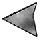# Phase Changes

## Phase Diagram: Part 4 EXPERIMENT G

### Triple Point

A line on a phase diagram represents conditions (T,P) under which two phases can coexist at equilibrium, that is, a phase transition occurs.

Inspection of the phase diagram shown below reveals an intersection of lines such that a single point, called the triple point, lies on three lines. The temperature and pressure at the triple point are denoted by T3 and P3, respectively. At the triple point three phases can coexist at equilibrium; thus, the fusion, vaporization, and sublimation equilibria all occur simultaneously.

The same phase diagram and cylinder containing the sample are shown below. As before, the solid phase is green in color, the liquid is blue, and the gas is red. A purple dot marks the current conditions.

1. Points A and B lies along an isobaric line with pressure P3. Select the "Point A" or "Point B" button to establish conditions on this line. Use the "Heat Sample" and "Cool Sample" button to move along this line through the triple point. Observe how all three phases can be found at the triple point.

2. Use the volume controls to very slightly increase or decrease the pressure so the current conditions lie just off the line connecting Points A and B. Once again heat or cool the sample to produce a phase change. How does the behavior in this case differ from that observed in part 1 above?

3. Points C and D lies along an isothermal line with temperature T3. Select the "Point C" or "Point D" button to establish conditions on this line. Use the "Increase Volume" or "Decrease Volume" button to move along this line through the triple point. Note that the blue line (representing the fusion reaction) rises nearly vertically but bends to the right. Thus after passing through the triple point while decreasing the volume, the stable phase is the solid. Observe the similarity between this behavior and that in Part 1.

4. What are the values of T3 and P3 for this substance?

 Phase Diagram Part 1 Part 2 Part 3 Part 4 Part 5Phase Changes Home Page